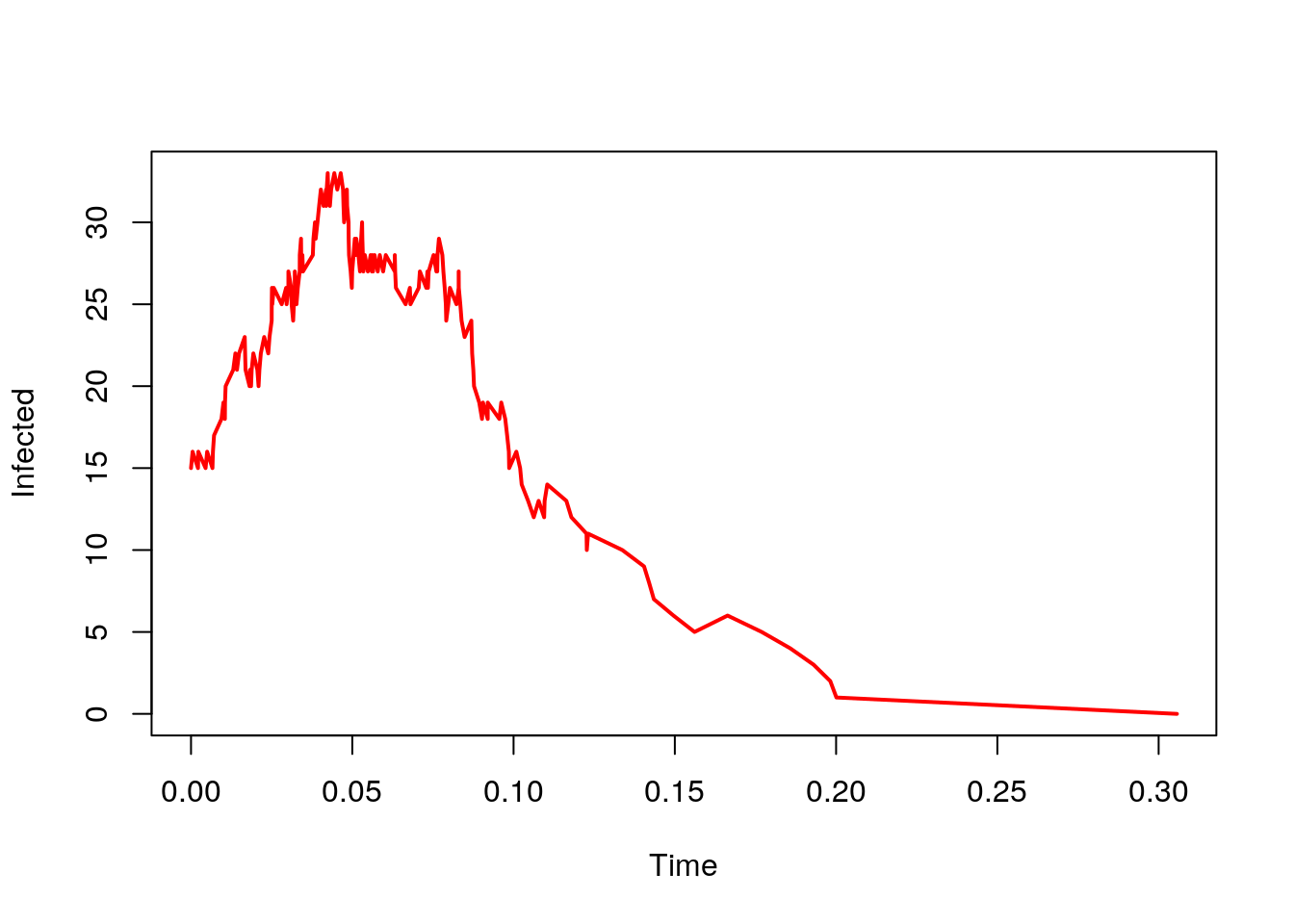## Introduction

In this exercise I demonstrate the use of Gillespie’s direct method for compartmental epidemic models.

My approach will be to wrap a function SIR.onestep (that generates both the next event time and event type) within another function that calls SIR.onestep iteratively until stopping criterion is reached.

Here is illustration for $$SIR$$ model without demography.

SIR.onestep <- function(x, params){
X <- x
Y <- x
Z <- x
N <- X+Y+Z
with(as.list(params),{
R <- c(beta*X*Y/N, gamma*Y)
E <- matrix(c(-1,1,0,
0,-1,1),
ncol=3, byrow=TRUE)
S <- -log(runif(1))/sum(R)   # Step 1 of Gillespie
U <- runif(1)
rescaledU <- U*sum(R)
m <- min(which(cumsum(R) >= rescaledU))
x <- x[2:4] + E[m,]
return(out <- c(S, x))
}
)
}

Here we write SIR.model to iteratively call SIR.onestep to simulate one epidemic. Note that because there is no demography, I had to put a break in to terminate the loop if the epidemic dies out. I did this with the code if (x == 0) break.

SIR.model <- function(x, params, nstep=1000){
output <- array(dim=c(nstep+1, 4))
colnames(output) <- c('Time', 'Susceptibles', 'Infected', 'Recovered')
output[1,] <- x
for(i in 1:nstep){
output[i+1,] <- x <- SIR.onestep(x, params)
if (x == 0) break
}
return(output)
}

Now, I can set up some initial conditions and simulate. At the end of this code chunk I remove any rows that are NA and convert to a data frame.

xstart <- c(time=0, X=100, Y=15, Z=0)
params <- list(beta=60, gamma=365/13)
result <- SIR.model(xstart, params, nstep=1000)
result <- as.data.frame(na.omit(result))

The variable Time is just the time increments. I calculate the cumulative sum of this to get chronological time.

t <- c(cumsum(result$Time)) Now I can plot the number of infected over time. plot(t, result$Infected, col='red', lwd=2, type='l',
xlab='Time',
ylab='Infected')